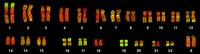# Finding a Motif in DNA solved by 21674

July 2, 2012, midnight by Rosalind Team

Topics: String Algorithms

## Combing Through the HaystackFigure 1. The human chromosomes stained with a probe for Alu elements, shown in green.

Finding the same interval of DNA in the genomes of two different organisms (often taken from different species) is highly suggestive that the interval has the same function in both organisms.

We define a motif as such a commonly shared interval of DNA. A common task in molecular biology is to search an organism's genome for a known motif.

The situation is complicated by the fact that genomes are riddled with intervals of DNA that occur multiple times (possibly with slight modifications), called repeats. These repeats occur far more often than would be dictated by random chance, indicating that genomes are anything but random and in fact illustrate that the language of DNA must be very powerful (compare with the frequent reuse of common words in any human language).

The most common repeat in humans is the Alu repeat, which is approximately 300 bp long and recurs around a million times throughout every human genome (see Figure 1). However, Alu has not been found to serve a positive purpose, and appears in fact to be parasitic: when a new Alu repeat is inserted into a genome, it frequently causes genetic disorders.

## Problem

Given two strings $s$ and $t$, $t$ is a substring of $s$ if $t$ is contained as a contiguous collection of symbols in $s$ (as a result, $t$ must be no longer than $s$).

The position of a symbol in a string is the total number of symbols found to its left, including itself (e.g., the positions of all occurrences of 'U' in "AUGCUUCAGAAAGGUCUUACG" are 2, 5, 6, 15, 17, and 18). The symbol at position $i$ of $s$ is denoted by $s[i]$.

A substring of $s$ can be represented as $s[j:k]$, where $j$ and $k$ represent the starting and ending positions of the substring in $s$; for example, if $s$ = "AUGCUUCAGAAAGGUCUUACG", then $s[2:5]$ = "UGCU".

The location of a substring $s[j:k]$ is its beginning position $j$; note that $t$ will have multiple locations in $s$ if it occurs more than once as a substring of $s$ (see the Sample below).

Given: Two DNA strings $s$ and $t$ (each of length at most 1 kbp).

Return: All locations of $t$ as a substring of $s$.

## Sample Dataset

GATATATGCATATACTT
ATAT


## Sample Output

2 4 10


## Note

Different programming languages use different notations for positions of symbols in strings. Above, we use 1-based numbering, as opposed to 0-based numbering, which is used in Python. For $s$ = "AUGCUUCAGAAAGGUCUUACG", 1-based numbering would state that $s$ = 'A' is the first symbol of the string, whereas this symbol is represented by $s$ in 0-based numbering. The idea of 0-based numbering propagates to substring indexing, so that $s[2:5]$ becomes "GCUU" instead of "UGCU".

Note that in some programming languages, such as Python, s[j:k] returns only fragment from index $j$ up to but not including index $k$, so that s[2:5] actually becomes "UGC", not "UGCU".